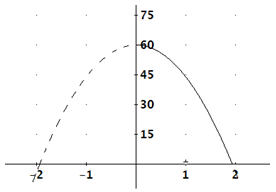Projectile Motion
Example 1

A construction worker accidentally drops a hammer from a building that is 60 feet above the concrete. Write an equation and make a graph to model the height of this object over time.

Step 1. Determine the initial height of the object and its initial velocity.

The initial height, s, is 60 feet. The initial velocity, v, is 0 since the object was dropped, not thrown.

Step 2. Substitute these values into the formula.

h(t) = -16t2 + 0t + 60

Step 3. Make the graph.*Note: The left side of the graph is dashed because the negative values of time are not part of the domain of the function. Also, the parabola stops at y = 0 to indicate the object does not go below ground.